Courses

# Capacitance Practice Level - 1

## 40 Questions MCQ Test Exclusive Video Lectures of Class 12 Physics by Experts | Capacitance Practice Level - 1

Description
This mock test of Capacitance Practice Level - 1 for Class 12 helps you for every Class 12 entrance exam. This contains 40 Multiple Choice Questions for Class 12 Capacitance Practice Level - 1 (mcq) to study with solutions a complete question bank. The solved questions answers in this Capacitance Practice Level - 1 quiz give you a good mix of easy questions and tough questions. Class 12 students definitely take this Capacitance Practice Level - 1 exercise for a better result in the exam. You can find other Capacitance Practice Level - 1 extra questions, long questions & short questions for Class 12 on EduRev as well by searching above.
QUESTION: 1

Solution:
QUESTION: 2

Solution:
QUESTION: 3

### A capacitor is charged by using a battery which is then disconnected. A dielectric slab is then slipped between the plates, which results in:

Solution:
QUESTION: 4

If K is the dielectric constant of a medium and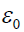permittivity of free space, then the energy stored per unit volume of the medium is given by:

Solution:
QUESTION: 5

If parallel plate capacitor has plates with area A and separation d. A battery charges the plates to a potential difference V0. The battery is then disconnected and a dielectric slab of thickness d is introduced. The ratio of energy stored in the capacitor before and after the slab is introduced, is:

Solution:
QUESTION: 6

The resultant capacitance between A and B in the Fig.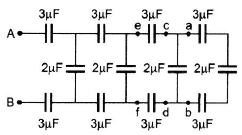Solution:
QUESTION: 7

In the given figure, three capacitors C1, C2 and C3 are joined to a battery, with symbols having their usual meanings, the correct conditions will be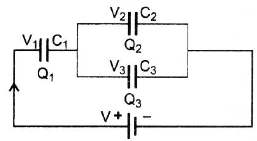Solution:
QUESTION: 8

n capacitors each having capacitance C and breakdown voltage V are joined in parallel. The capacitance and the breakdown voltage of the combination are:

Solution:
QUESTION: 9

An infinite number of identical capacitors each of capacitance 1 μF are connected as shown in the figure. Then the equivalent capacitance between A and B is: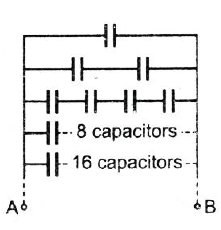Solution:
QUESTION: 10

Two identical parallel plate capacitors are placed in series and connected to a constant voltage source of V0 volt. If one of the capacitors is completely immersed in a liquid with dielectric constant K, the potential difference between the plates of the other capacitor will change to:

Solution:
QUESTION: 11

Six equal capacitors each of capacitance C are connected as shown in the figure. Then the equivalent capacitance between A and B is: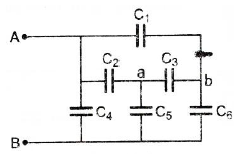Solution:
QUESTION: 12

A battery of emf V volt, resistors R1 and R2, a condenser C and switches S1 and S2 are connected in the circuit as shown in the figure below: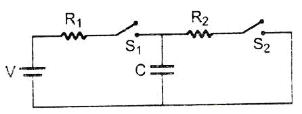Solution:
QUESTION: 13

Two identical parallel plate capacitors of same dimensions joined in series are connected to a DC source. When one of the plates of one capacitor is brought closer to the other plate:

Solution:
QUESTION: 14

A parallel plate condenser with plate area A and separation d is filled with two dielectric materials as shown in the given below. The dielectric constants are K1 and K2 respectively. The capacitance will be: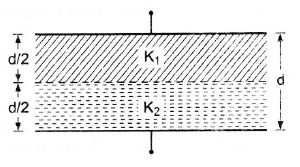Solution:
QUESTION: 15

A circuit has a section AB as shown in the figure. If the potential difference A between points A and B is V volt, then potential difference across C1 is: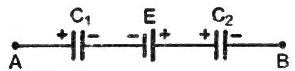Solution:
QUESTION: 16

For the circuit shown in the figure, the charge on 4μF capacitor is: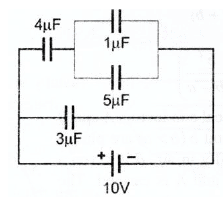Solution:
QUESTION: 17

Two capacitors, 3μF and 4μF, are individually charged across a 6 volt battery. After being disconnected from the battery they are connected together with the negative plate of one attached to the positive plate of the other. What is the common potential?

Solution:
QUESTION: 18

The plates of a parallel plate capacitor are charged up to 100 volt. A 2 mm thick plate is inserted between the plates, then to maintain the same potential difference, the distance between the capacitor plates is increased by 1.6 mm. The dielectric constant of the plate is:

Solution:
QUESTION: 19

A capacitor of capacitance 1μF withstands a maximum voltage of 6 kV, while another capacitor of capacitance 2μF, the maximum voltage 4 kV. If they are connected in series, the combination can withstand a maximum of

Solution:
QUESTION: 20

Seven capacitors each of capacitance 2μF are to be connected to obtain a capacitance of (10/11) μF. Which of the following combinations is possible?

Solution:
QUESTION: 21

Condenser A has a capacity of 15 μF when it is filled with a medium of dielectric constant 15. Another condenser B has a capacity of 1μF with air between the plates. Both are charged separately by a battery of 100 V. After charging, both are connected in parallel without the battery and the dielectric material being removed. The common potential is now:

Solution:
QUESTION: 22

Four identical capacitors are connected in series with a 10 V battery as shown. The point N is earthed. The potentials of points A and B are: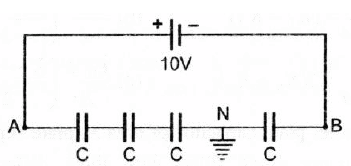Solution:
QUESTION: 23

A parallel plate capacitor with air as medium between the plates has a capacitance of 10μF. The area of the capacitor is divided into two equal halves and filled with two media having dielectric constants K1 = 2 and K2 = 4. The capacitance of the system will now be:

Solution:
QUESTION: 24

A parallel plate capacitor with a dielectric slab (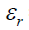= 3) filling the space between the plates is charged to potential V and isolated. Then the dielectric slab is drawn out and another dielectric of equal thickness but= 2 is introduced between the plates. The ratio of the energy stored in the capacitor later to that initially is:

Solution:
QUESTION: 25

You are given 32 capacitors each having capacity 4 μF. How do you connect all of them to prepare a composite capacity having a capacitance 8μF?

Solution:
QUESTION: 26

A parallel plate condenser is filled with two dielectrics as shown in figure. Area of each plate is A metre2 and the separation is d metre. The dielectric constants are K1 and K2 respectively. Its capacitance in farad will be: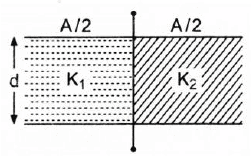Solution:
QUESTION: 27

A parallel plate capacitor of area A, plate separation d and capacitance C is filled with three different dielectric materials having dielectric constants K1, K2 and K3 as shown. If a single dielectric material is to be used to have the same capacitance C in this capacitor, then its dielectric constant K is given by: (A = Area of plates)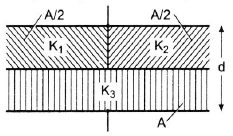Solution:
QUESTION: 28

Two spherical conductors A and B of radii a and b (b > a) are placed concentrically in air. A is given a charge +Q while B is earthed. Then the equivalent capacitance of the system is: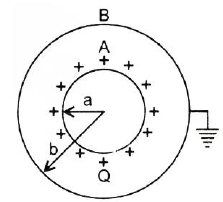Solution:
QUESTION: 29

Two spherical conductors A and B of radii a and b(b > a) are placed concentrically in air. B is given a charge +Q and A is earthed. The equivalent capacitance of the system is :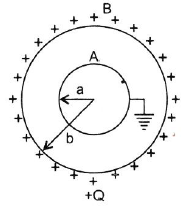Solution:
QUESTION: 30

Two spherical conductors A and B of radii a and b (b > a) are placed concentrically in air. The two are connected by a copper wire as shown in figure. Then the equivalent capacitance of the system is: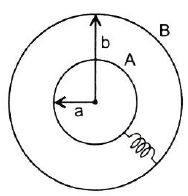Solution:
QUESTION: 31

Four metallic plates, each with a surface area of one side A, are placed at a distance d from each other. The plates are connected as shown in the adjoining figure. Then the capacitance of the system between a and b is: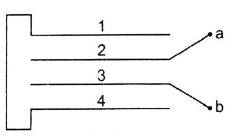Solution:
QUESTION: 32

The plates of a capacitor are charged to potential difference of V volts and then connected across a resistor. The potential difference across the capacitor decreases exponentially with respect to time. After one second, the potential difference between the plates is V/3; then after two seconds from the start, the potential difference between the plates is:

Solution:
QUESTION: 33

Five capacitors of 10μF capacity each are connected to a DC potential of 100 volts as shown in figure. The equivalent capacitance between the points A and B will be equal to: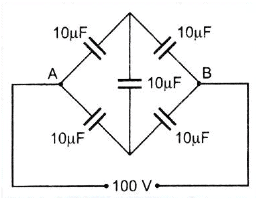Solution:
QUESTION: 34

Four metallic plates, each with surface area of one side A, are placed at a distance d from each other. The plates are connected as shown in the adjoining figure. Then the capacitance of the system between P and Q is: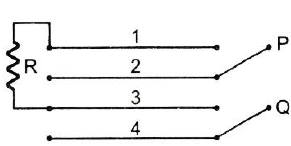Solution:
QUESTION: 35

Four capacitors are connected in a circuit as shown in figure. The effective capacitance between points A and B will be: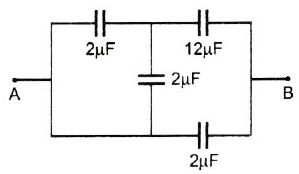Solution:
QUESTION: 36

Three plates A, B, C each of area 50 cm2 have separation 3 mm between A and B and 3 mm between B and C. The energy stored when the plates are fully charged is: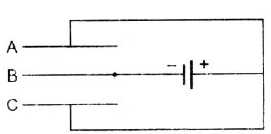Solution:
QUESTION: 37

The plates of a capacitor are charged to a potential difference of 320 volt and are then connected to a resistor. The potential difference across the capacitor decays exponentially with time. After 1 sec, the potential difference between the plates of the capacitor is 240 V, then after 2 and 3 seconds the potential difference between the plates will be, respectively:

Solution:
QUESTION: 38

An uncharged parallel plate capacitor having a dielectric of constant K is connected to a similar air-cored parallel capacitor charged to a potential V. The two share the charge and the common potential is V'. The dielectric constant K is:

Solution:
QUESTION: 39

A parallel plate capacitor is connected to a battery. The plates are pulled apart with a uniform speed v. If x is the separation between the plates, then the time rate of change of the electrostatic energy of the condenser is proportional to:

Solution:
QUESTION: 40

Two identical air-filled parallel plate capacitors are charged to the same potential in the manner shown by closing the switch S. If now the switch S is opened and the space between the plates filled with a dielectric of specific inductive capacity or relative permittivity 3, then: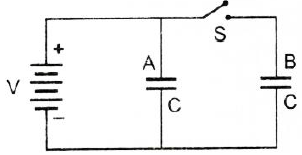Solution: